# US6373906B1 - Method and apparatus for Viterbi detection of generalized partial response signals including two-way add/compare/select for improved channel speed - Google Patents

Method and apparatus for Viterbi detection of generalized partial response signals including two-way add/compare/select for improved channel speed Download PDF

## Info

Publication number
US6373906B1
US6373906B1 US09/768,802 US76880201A US6373906B1 US 6373906 B1 US6373906 B1 US 6373906B1 US 76880201 A US76880201 A US 76880201A US 6373906 B1 US6373906 B1 US 6373906B1
Authority
US
United States
Prior art keywords
compare
way
speed
metric
state metric
Prior art date
Legal status (The legal status is an assumption and is not a legal conclusion. Google has not performed a legal analysis and makes no representation as to the accuracy of the status listed.)
Active
Application number
US09/768,802
Inventor
Roy Daron Cideciyan
Jonathan Darrel Coker
Evangelos S. Eleftheriou
Richard Leo Galbraith
Allen Prescott Haar
Frank Ray Keyser, III
David James Stanek
Current Assignee (The listed assignees may be inaccurate. Google has not performed a legal analysis and makes no representation or warranty as to the accuracy of the list.)
Western Digital Technologies Inc
Original Assignee
Priority date (The priority date is an assumption and is not a legal conclusion. Google has not performed a legal analysis and makes no representation as to the accuracy of the date listed.)
Filing date
Publication date
Priority to US09/697,467 priority Critical patent/US6377635B1/en
Application filed by International Business Machines Corp filed Critical International Business Machines Corp
Priority to US09/768,802 priority patent/US6373906B1/en
Assigned to INTERNATIONAL BUSINESS MACHINES CORPORATION reassignment INTERNATIONAL BUSINESS MACHINES CORPORATION ASSIGNMENT OF ASSIGNORS INTEREST (SEE DOCUMENT FOR DETAILS). Assignors: HAAR, ALLEN PRESCOTT, KEYSER III, FRANK RAY, CIDECIYAN, ROY DARON, COKER, JONATHAN DARREL, ELEFTHERIOU, EVANGELOS S., GALBRAITH, RICHARD LEO, STANEK, DAVID JAMES
Application granted granted Critical
Publication of US6373906B1 publication Critical patent/US6373906B1/en
Assigned to HITACHI GLOBAL STORAGE TECHNOLOGIES NETHERLANDS B.V. reassignment HITACHI GLOBAL STORAGE TECHNOLOGIES NETHERLANDS B.V. CHANGE OF NAME (SEE DOCUMENT FOR DETAILS). Assignors: MARIANA HDD B.V.
Assigned to MARIANA HDD B.V. reassignment MARIANA HDD B.V. ASSIGNMENT OF ASSIGNORS INTEREST (SEE DOCUMENT FOR DETAILS). Assignors: INTERNATIONAL BUSINESS MACHINES CORPORATION
Assigned to HGST Netherlands B.V. reassignment HGST Netherlands B.V. CHANGE OF NAME (SEE DOCUMENT FOR DETAILS). Assignors: HITACHI GLOBAL STORAGE TECHNOLOGIES NETHERLANDS B.V.
Assigned to WESTERN DIGITAL TECHNOLOGIES, INC. reassignment WESTERN DIGITAL TECHNOLOGIES, INC. ASSIGNMENT OF ASSIGNORS INTEREST (SEE DOCUMENT FOR DETAILS). Assignors: HGST Netherlands B.V.
Application status is Active legal-status Critical
Anticipated expiration legal-status Critical

• 230000036961 partial Effects 0 abstract claims description title 89
• 230000004044 response Effects 0 abstract claims description title 49
• 230000001976 improved Effects 0 abstract claims description title 14
• 239000011701 zinc Substances 0 abstract claims description 17
• 230000001419 dependent Effects 0 claims description 35
• 230000001131 transforming Effects 0 description 15
• 230000000875 corresponding Effects 0 description 10
• 238000007476 Maximum Likelihood Methods 0 description 7
• 230000001721 combination Effects 0 description 7
• 230000002087 whitening Effects 0 description 7
• 241001442055 Vipera berus Species 0 description 5
• 238000007493 shaping process Methods 0 description 5
• 238000000034 methods Methods 0 description 3
• 238000005311 autocorrelation function Methods 0 description 2
• 238000003860 storage Methods 0 description 2
• 238000000844 transformation Methods 0 description 2
• 238000007792 addition Methods 0 description 1
• 238000004891 communication Methods 0 description 1
• 230000000694 effects Effects 0 description 1
• 230000015654 memory Effects 0 description 1
• 238000005457 optimization Methods 0 description 1
• 230000002829 reduced Effects 0 description 1
• 230000001603 reducing Effects 0 description 1
• 238000006722 reduction reaction Methods 0 description 1
• 230000011664 signaling Effects 0 description 1

## Images

•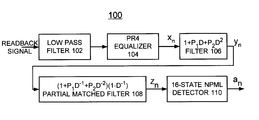•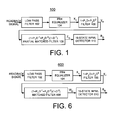•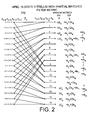•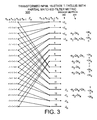•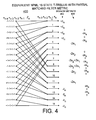•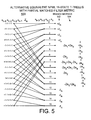•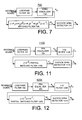•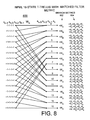•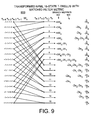•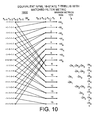•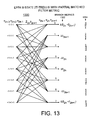•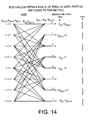•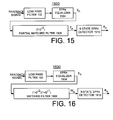•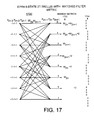•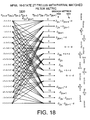•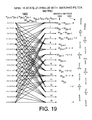••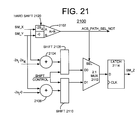## Classifications

• HELECTRICITY
• H04ELECTRIC COMMUNICATION TECHNIQUE
• H04LTRANSMISSION OF DIGITAL INFORMATION, e.g. TELEGRAPHIC COMMUNICATION
• H04L1/00Arrangements for detecting or preventing errors in the information received
• H04L1/004Arrangements for detecting or preventing errors in the information received by using forward error control
• H04L1/0045Arrangements at the receiver end
• H04L1/0054Maximum-likelihood or sequential decoding, e.g. Viterbi, Fano, ZJ algorithms
• HELECTRICITY
• H04ELECTRIC COMMUNICATION TECHNIQUE
• H04LTRANSMISSION OF DIGITAL INFORMATION, e.g. TELEGRAPHIC COMMUNICATION
• H04L25/00Baseband systems
• H04L25/38Synchronous or start-stop systems, e.g. for Baudot code
• H04L25/40Transmitting circuits; Receiving circuits
• H04L25/49Transmitting circuits; Receiving circuits using code conversion at the transmitter; using predistortion; using insertion of idle bits for obtaining a desired frequency spectrum; using three or more amplitude levels ; Baseband coding techniques specific to data transmission systems
• H04L25/497Transmitting circuits; Receiving circuits using code conversion at the transmitter; using predistortion; using insertion of idle bits for obtaining a desired frequency spectrum; using three or more amplitude levels ; Baseband coding techniques specific to data transmission systems by correlative coding, e.g. partial response coding or echo modulation coding transmitters and receivers for partial response systems

## Abstract

Apparatus is provided for implementing high-speed and area efficient architectures for Viterbi detection of generalized partial response signals including two-way add/compare/select for improved channel speed. The two-way add/compare/select includes a two-way compare for comparing first and second state metric input values and a pair of two-way adds in parallel with the two-way compare for respectively adding the first and second state metric input values with a second input value. The second input value includes a time varying term or a constant term. The time varying terms are expressed as outputs Zn of a partial matched filter or as outputs Wn of a matched filter. A multiplexer is coupled to the pair of two-way adds, the multiplexer receiving a selectable input controlled by the two-way compare. A pair of shifts coupled between the pair of two-way adds and the multiplexer receive a shift control input for providing metric bounding to avoid underflow. The two-way compare for comparing first and second state metric input values can include a hard shift for providing an add for the first state metric input value and then a compare between a resultant first state metric input value and the second state metric input value.

## Description

CROSS REFERENCE TO RELATED APPLICATION

This application is a continuation-in-part of patent application Ser. No. 09/697,467 filed on Oct. 26, 2000. The above-identified application is incorporated herein by reference.

FIELD OF THE INVENTION

The present invention relates generally to data detection methods and apparatus for generalized partial-response channels in a direct access storage device, and more particularly, relates to a general methodology and apparatus for implementing high-speed and area efficient architectures for Viterbi detection of generalized partial response signals using transformed metrics, such as the partial matched filter branch metrics and the matched filter branch metrics and including two-way add/compare/select for improved channel speed.

DESCRIPTION OF THE RELATED ART

Partial-response signaling with maximum-likelihood sequence detection techniques are known for digital data communication and recording applications. U.S. Pat. No. 5,619,539 discloses data detection methods and apparatus for a direct access storage device including an 8-state extended partial-response class 4 (EPR4) trellis with modified branch metrics based upon heuristics so that the number of nonzero trellis branch constants is reduced.

U.S. Pat. No. 5,430,744 discloses a Viterbi decoder having a recursive processor modified to process each node in a trellis of a partial-response coded signal via heuristics to shift the branch metric additions over the node to effectuate compare, select, add operation order on the predecessor survivor metrics terminating in that node, to compare the metrics of the predecessor sequences terminating in the node, to select a survivor sequence, and to add the shifted branch metrics to the metric of the selected survivor sequence.

A need exists for a methodology for implementing high-speed and area efficient architectures for Viterbi detection of generalized partial-response signals. It is desirable to provide a general methodology and apparatus for implementing high-speed and area efficient architectures for Viterbi detection of generalized partial response signals using partial matched filter branch metrics and matched filter branch metrics.

SUMMARY OF THE INVENTION

A principal object of the present invention is to provide a methodology for implementing high-speed and area efficient architectures for Viterbi detection of generalized partial response signals including two-way add/compare/select for improved channel speed. Other important objects of the present invention are to provide Viterbi detectors for generalized partial response signals including two-way add/compare/select for improved channel speed substantially without negative effect and that overcome many of the disadvantages of prior art arrangements.

In brief, apparatus is provided for implementing high-speed and area efficient architectures for Viterbi detection of generalized partial response signals including two-way add/compare/select for improved channel speed. The two-way add/compare/select includes a two-way compare for comparing first and second state metric input values and a pair of two-way adds in parallel with the two-way compare for respectively adding the first and second state metric input values with a second input value.

In accordance with features of the invention, the second input value includes a time varying term or a constant term. The time varying terms are expressed as outputs Zn of a partial matched filter or as outputs Wn of a matched filter. A multiplexer is coupled to the pair of two-way adds, the multiplexer receiving a selectable input controlled by the two-way compare. A pair of shifts coupled between the pair of two-way adds and the multiplexer receive a shift control input for providing metric bounding to avoid underflow. The two-way compare for comparing first and second state metric input values can include a hard shift for providing an add for the first state metric input value and then a compare between a resultant first state metric input value and the second state metric input value.

BRIEF DESCRIPTION OF THE DRAWINGS

The present invention together with the above and other objects and advantages may best be understood from the following detailed description of the preferred embodiments of the invention illustrated in the drawings, wherein:

FIG. 1 is a block diagram representation illustrating a generalized partial-response data channel including a NPML 16-state detector for PR4 equalized signals using partial matched filter metric in accordance with the preferred embodiment;

FIG. 2 illustrates a NPML 16-state trellis with partial matched filter metric in accordance with the preferred embodiment;

FIG. 3 illustrates a transformed NPML 16-state trellis with partial matched filter metric in accordance with the preferred embodiment;

FIG. 4 illustrates an equivalent NPML 16-state trellis with partial matched filter metric in accordance with the preferred embodiment;

FIG. 5 illustrates another equivalent NPML 16-state trellis with partial matched filter metric in accordance with the preferred embodiment;

FIG. 6 is a block diagram representation illustrating a generalized partial-response data channel including a NPML 16-state detector for PR4 equalized signals using matched filter metric in accordance with the preferred embodiment;

FIG. 7 illustrates a NPML 16-state detector for EPR4 equalized signals using matched filter metric in accordance with the preferred embodiment;

FIG. 8 illustrates a NPML 16-state trellis with matched filter metric in accordance with the preferred embodiment;

FIG. 9 illustrates a transformed NPML 16-state trellis with matched filter metric in accordance with the preferred embodiment;

FIG. 10 illustrates an equivalent NPML 16-state trellis with matched filter metric in accordance with the preferred embodiment;

FIG. 11 illustrates is a block diagram representation illustrating a generalized partial-response data channel including a EPR4 detector for PR4 equalized signals using matched filter metric in accordance with the preferred embodiment;

FIG. 12 illustrates is a block diagram representation illustrating a generalized partial-response data channel including an EPR4 detector for PR4 equalized signals using matched filter metric in accordance with the preferred embodiment;

FIG. 13 illustrates an EPR4 2T trellis with partial matched filter metric in accordance with the preferred embodiment;

FIG. 14 illustrates an equivalent EPR4 2T trellis with partial matched filter metric in accordance with the preferred embodiment;

FIG. 15 illustrates is a block diagram representation illustrating a generalized partial-response data channel including an EPR4 detector for EPR4 equalized signals using partial matched filter metric in accordance with the preferred embodiment;

FIG. 16 illustrates is a block diagram representation illustrating a generalized partial-response data channel including an EPR4 detector for EPR4 equalized signals using matched filter metric in accordance with the preferred embodiment;

FIG. 17 illustrates an EPR4 2T trellis with matched filter metric in accordance with the preferred embodiment;

FIG. 18 illustrates a NPML 16-state 2T trellis with partial matched filter metric in accordance with the preferred embodiment;

FIG. 19 illustrates a NPML 16-state 2T trellis with matched filter metric in accordance with the preferred embodiment; and

FIGS. 20 and 21 illustrate add compare select (ACS) units in accordance with the preferred embodiment.

DETAILED DESCRIPTION OF THE PREFERRED EMBODIMENTS

In accordance with features of the invention, a general methodology for implementing high-speed and area efficient architectures for Viterbi detection of generalized partial-response signals is provided. Optimization of the Viterbi detector architecture achieves substantial reduction in hardware complexity and power consumption. The conventional Viterbi detector operating on an arbitrary generalized partial-response target with L coefficients requires 2L states with 2L+1 branch metrics of the form ayn+c where the constants a and c are not necessarily the same for each trellis branch.

In accordance with features of the invention, the branch metrics are transformed so that some of the constant terms and all of the data dependent or time-varying terms are shifted after the add/compare/select (ACS) unit and added directly to the state metrics. There are only 2L−1 additions with data dependent or time-varying terms and at most 2L−1 additions with constant terms after the add/compare/select (ACS) units.

Furthermore, the branch metrics themselves become constants. There are only 2L−1 non-zero constants as branch metrics.

In accordance with features of the invention, it is shown that the state metrics of a Viterbi detector possess a time-invariance property which together with the distributive law min(a+x,b+x)=min(a,b)+x allows to shift the data dependent or time-varying terms of the branch metrics to the output of the add-compare-select (ACS) units and to also minimize the total number of non-zero constants on the trellis branches. For a given generalized partial response target the time-invariance property guarantees how to systematically find the minimum number of non-zero constants associated with the branch adders without resorting to heuristics. Furthermore, it provides a method for exhaustively determining all possible realizations of the data dependent or time-varying terms of the branch metrics, expressed as outputs of matched-filters or partial matched-filters. The time-invariance property also holds for Viterbi detectors that process multiple samples per trellis-branch thus allowing implementations at any desired speed. The application of the general methodology is illustrated with specific embodiments related to noise-predictive maximum-likelihood (NPML) or “fractional coefficient” detection with targets of the form (1−D2)(1+p1D+p2D2) where p1 and p2 are arbitrary real numbers, not necessarily integers, with the corresponding detectors operating on a T or 2T basis, and extended partial response class 4 (EPR4) targets with a Viterbi detector operating on a 2T basis, where 1/T is the sample rate.

Consider a generalized partial response (PR) shaped signal in the presence of noise, that is: $\begin{array}{cc}{y}_{n}={a}_{n}+\sum _{i=1}^{L}{f}_{i}{a}_{n-i}+{\eta }_{n}& \left(1\right)\end{array}$

where an is the data symbol at time nT, F(D)=(1+f1D+f2D2+ . . . +fLDL) is the generalized PR polynomial and an is the noise sample at the input of the detector. The Viterbi detector operating on yn will find the sequence {ân} that minimizes the following metric: $\begin{array}{cc}J=\sum _{n}{\left\{{y}_{n}-\left({a}_{n}+\sum _{i=1}^{L}{f}_{i}{a}_{n-i}\right)\right\}}^{2}& \left(2\right)\end{array}$

or equivalently minimize the metric: $\begin{array}{cc}J=-2\sum _{n}{y}_{n}\left({a}_{n}+\sum _{i=1}^{L}{f}_{i}{a}_{n-i}\right)+\sum _{n}{\left({a}_{n}+\sum _{i=1}^{L}{f}_{i}{a}_{n-i}\right)}^{2}& \left(3\right)\end{array}$

For saturation recording systems the channel input an is binary, that is +1 or −1. In this case the metric in equation (3) is simplified to $\begin{array}{cc}\begin{array}{c}J=\text{ }-\sum _{n}{y}_{n}\left({a}_{n}+\sum _{i=1}^{L}{f}_{i}{a}_{n-i}\right)+\\ \text{ }\sum _{n}\left(\sum _{i=1}^{L}{f}_{i}{a}_{n}{a}_{n-i}+\sum _{i=1}^{L}\sum _{j>i}^{L}{f}_{i}{f}_{j}{a}_{n-i}{a}_{n-j}\right)\end{array}& \left(4\right)\end{array}$

where the first sum contains terms that depend on the channel output, referred to as data dependent or time-varying terms, and the second sum contains terms that do not depend on the channel output, referred to as constants. The following property is crucial in simplifying the architecture/implementation of the Viterbi detector. Observe that in general: $\begin{array}{cc}\sum _{n=0}^{\infty }{y}_{n}{a}_{n-i}=\sum _{n=-i}^{\infty }{y}_{n+i}{a}_{n}=\sum _{n=0}^{\infty }{y}_{n+i}{a}_{n}& \left(5\right)\end{array}$

is valid, where the last equality holds because of causality, i.e., an=0 for n<0. The property stated in equation (5) is called the “time-invariance” property of the state metrics. Note that this property can be independently applied to the two summation terms in equation (4). For a channel memory of L symbols, equation (5) suggests that there are L1 possible sets of data dependent or time-varying branch metric terms and $\prod _{i=0}^{L-1}{\left(L-i\right)}^{L-i}$

possible sets of branch constants within the window an, . . . an−L. In order to optimize the Viterbi detector architecture with respect to speed and area one has to identify the set of data dependent or time-varying branch metric terms that shifts after the ACS unit and the set of branch metric constants with the maximum number of zeros. Since the set of all possible combinations is well defined and finite, the desired set of constants with the maximum number of zeros can be found, for example, via a computer search.

Consider now matched filter and partial matched filter transformations. Applying the time-invariance property to the first summation term in equation (4) one obtains: $\begin{array}{cc}\begin{array}{c}J=\text{ }-\sum _{n}{a}_{n}\left({y}_{n}+\sum _{i=1}^{L}{f}_{i}{y}_{n+i}\right)+\\ \text{ }\sum _{n}\left(\sum _{i=1}^{L}{f}_{i}{a}_{n}{a}_{n-i}+\sum _{i=1}^{L-1}\sum _{j>i}^{L}{f}_{i}{f}_{j}{a}_{n-i}{a}_{n-j}\right)\end{array}& \left(6\right)\end{array}$

Let $\begin{array}{cc}{W}_{n}={y}_{n}+\sum _{i=1}^{L}{f}_{i}{y}_{n+i}& \left(7\right)\end{array}$

Clearly, Wn represents the output of a filter matched to the generalized PR channel polynomial F(D). Substituting equation (7) into equation (6) we obtain: $\begin{array}{cc}J=-\sum _{n}{a}_{n}{W}_{n}+\sum _{n}\left(\sum _{i=1}^{L}{f}_{i}{a}_{n}{a}_{n-i}+\sum _{i=1}^{L}\sum _{j>i}^{L}{f}_{i}{f}_{j}{a}_{n-i}{a}_{n-j}\right)& \left(8\right)\end{array}$

The above transformed metric achieves that the channel output dependent term of the branch metric, i.e., an Wn can be shifted after the ACS unit leaving on the trellis branches only constants.

Consider, as a second example, a generalized PR polynomial of the following form:

F(D)=(1−D 2)(1+p 1 D+p 2 D 2 + . . . +p L−2 D L−2)=(1−D 2)P(D)  (9)

where the coefficients p1,p2, . . . pL−2 are usually selected so that the noise at the input of the detector is as white as possible. In this case the coefficients p1,p2, . . . pL−2 are the predictor coefficients and the polynomial P(D) is the whitening filter of order L−2. In this example let $\begin{array}{cc}{Z}_{n}={y}_{n}+\sum _{i=1}^{L-2}{p}_{i}{y}_{n+i}& \left(10\right)\end{array}$

denote the output of a filter which is matched only to the filter P(D), so that it is partially matched to the channel polynomial F(D). Using the time-invariance property stated in equation (5) and the partial matched-filter signal Zn the following equivalent metric is obtained: $\begin{array}{cc}\begin{array}{c}J=\text{ }-\sum _{n}\left({a}_{n}-{a}_{n-2}\right){Z}_{n}+\\ \text{ }\sum _{n}\left(\sum _{i=1}^{L}{f}_{i}{a}_{n}{a}_{n-i}+\sum _{i=1}^{L-1}\sum _{j>i}^{L}{f}_{i}{f}_{j}{a}_{n-i}{a}_{n-j}\right)\end{array}& \left(11\right)\end{array}$

As in the matched-filter case, the transformed metric in equation (11) achieves that the channel output dependent term of the branch metric, that is, Zn(an−an−2) can be shifted after the ACS units leaving on the trellis branches only constants. Clearly, there are other partial matched-filter transformations that can be applied to the metric in equation (6) such as, (1−D)P(D), (1+D)P(D), and the like.

Applying the time-invariance property to the second summation term in equation (8) so that an comes out as a common factor we obtain the equivalent metric: $\begin{array}{cc}J=-\sum _{n}{a}_{n}{W}_{n}+\sum _{n}\left({a}_{n}\sum _{i=1}^{L}{s}_{i}{a}_{n-i}\right)& \left(12\right)\end{array}$

where si, i=1, 2, . . . , L are the one sided coefficients of the autocorrelation function of the generalized PR polynomial F(D), that is: $\begin{array}{cc}{s}_{i}={f}_{i}+\sum _{j=1}^{i+j\le L}{f}_{j}{f}_{i+j},\text{ }i=1,2,\dots \text{ },L& \left(13\right)\end{array}$

This particular metric in equation (12) is known in the literature as the matched filter metric.

In accordance with preferred embodiments for T-processing, for NPML or “fractional-target” 16-state detection with two predictor coefficients the target function is:

F(D)=(1−D 2)(1+p 1 D+p 2 D 2)=(1−D 2)P(D)  (14)

Let S(D)=F(D)F(D−1) be the D-transform of the autocorrelation function of the impulse response associated with the detector target. Then

S(D)=s 4 D −4+ s 3 D −3+ s 2 D −2+ s 1 D −1+ s 0 + s 1 D 1+ s 2 D 2+ s 3 D 3+ s 4 D 4  (15)

where,

s 1 =p 1(p 2+1),

s 2=−(1−p 2)2 −p 1 2

s 3 =−p 1(p 2+1),

s 4 =−p 2  (16)

In this case by applying the time-invariance transformation, the following equivalent matched-filter metric is obtained $\begin{array}{cc}\begin{array}{c}J=\text{ }-\sum _{n}\left({a}_{n}-{a}_{n+1}\right){Z}_{n}+\sum _{n}\left\{{s}_{1}{a}_{n}{a}_{n-1}+\left({s}_{2}+{s}_{4}\right){a}_{n}{a}_{n-2}-\\ \text{ }{s}_{1}{a}_{n}{a}_{n-3}+{s}_{4}{a}_{n}{a}_{n-4}-{s}_{1}{a}_{n-1}{a}_{n-2}+{s}_{1}{a}_{n-2}{a}_{n-3}-\\ \text{ }{s}_{4}{a}_{n-2}{a}_{n-4}+{s}_{4}\right\}\end{array}& \left(17\right)\end{array}$

Having reference now to the drawings, in FIG. 1, there is shown a generalized partial-response data channel of the preferred embodiment generally designated by the reference character 100. As shown in FIG. 1, a readback signal is applied to a low pass filter 102 and applied to a PR4 equalizer 104. The PR4 equalizer 104 is followed by a whitening/predictor filter 1+p1D+p2D2 106, a partial matched filter (131 D−1)(1+p1D−1+p2D−2) 108, and a corresponding 16-state noise-predictive maximum-likelihood (NPML) detector 110. The output of PR4 equalizer 104 is an equalized signal xn. The output of the whitening/predictor filter 1+p1D+p2D2 106 is the whitened output yn. The partial matched-filter (1−D−1)(1+p1D−1+p2D−2) 108 facilitates the transformation of the trellis according to the metric in equation (17) providing an output Zn. The 16-state NPML detector 110 provides output decisions an.

FIG. 2 illustrates a NPML 16-state trellis with partial matched filter metric in accordance with the preferred embodiment generally designated by the reference character 200. Transitioning through the trellis 200 from time nT represented by the nodes on the left hand side of the trellis to time (n+1)T represented by the nodes on the right hand side of the trellis, Add-Compare-Select (ACS) operations are performed to find the updated state metric. As shown in FIG. 2, NPML 16-state trellis with partial matched filter metric 200 includes 16 nodes or states and 32 branches connecting the 16 states together between time steps. The values an−4an−3an−2an−1 and an−3an−2an−1an are the trellis states (0-15) at times nT and (n+1)T, respectively. On the right side of the trellis, branch metric data dependent terms (+2Zn, −2Zn) 202 and branch metric constant terms (combinations of s1, s2, s4) 204 are shown. The output Zn of partial matched-filter (1−D−1)(1+p1D−1+p2D−2) 108 is shown above the trellis.

FIG. 3 illustrates a transformed NPML 16-state trellis with partial matched filter metric in accordance with the preferred embodiment generally designated by the reference character 300. As shown in FIG. 3, the NPML 16-state trellis with partial matched filter metric 200 of FIG. 2 is transformed by subtracting the constant term s2+2s4 from all the state metrics and normalizing all metric components by a factor of two. The resulting branch metric data dependent terms (+Zn, −Zn) 302 and branch metric constant terms (combinations of s1, s2, s4) 304 are shown on the right side of the trellis 300.

Referring to FIGS. 4 and 5, FIG. 4 illustrates an equivalent NPML 16-state trellis with partial matched filter metric in accordance with the preferred embodiment generally designated by the reference character 400. FIG. 4 shows the 16-state trellis 400 where the common data dependent terms and constant terms have been collected at the output of the ACS units by applying the distributive law min(a+x, b+x)=min(a,b)+x. FIG. 5 illustrates another equivalent NPML 16-state trellis with partial matched filter metric in accordance with the preferred embodiment generally designated by the reference character 500. Both trellises 400 and 500 require only 8 branch adders instead of 32 for the branch metric data dependent terms (+Zn, −Zn) 302. Furthermore, there are 4 additions with the branch metric data dependent terms +Zn 402, 502, 4 additions with the branch metric data dependent terms −Zn 402, 502, and 6 additions with branch metric constant terms 404, 504 following the ACS unit that involve only three constants, s1, s2, s4.

An equivalent metric realization can be obtained by applying a full matched-filter transformation to the metric in equation (4). By applying the time-invariance transformation we obtain the following equivalent matched-filter metric: $\begin{array}{cc}J=-\sum _{n}{a}_{n}{W}_{n}+\sum _{n}\left({a}_{n}\left(\sum _{i=1}^{4}{s}_{i}{a}_{n-i}\right)& \left(18\right)\end{array}$

FIG. 6 is a block diagram representation illustrating a generalized partial-response data channel including a NPML 16-state detector for PR4 equalized signals using matched filter metric in accordance with the preferred embodiment generally designated by the reference character 600. As shown in FIG. 6, a readback signal is applied to a low pass filter 102 and applied to a PR4 equalizer 104. The PR4 equalizer 104 is followed by a whitening/predictor filter 1+p1D+p2D2 106, a matched filter (1+p1D−1+p2D−2)(1−D−2) 608, and a corresponding 16-state noise-predictive maximum-likelihood (NPML) detector 610. The output of PR4 equalizer 104 is an equalized signal xn. The output of the whitening/predictor filter 1+p1D+p2D2 106 is the whitened output yn. The matched-filter (1+p1D−1+p2D−2) (1−D−2) 108 facilitates the transformation of the trellis according to the metric in equation (18) providing an output Wn. The 16-state NPML detector 610 provides output decisions an.

FIG. 7 illustrates a NPML 16-state detector for extended PR4 (EPR4) equalized signals using matched filter metric in accordance with the preferred embodiment generally designated by the reference character 700. As shown in FIG. 7, a readback signal is applied to a low pass filter 102 and applied to an EPR4 equalizer 704. The EPR4 equalizer 704 replaces the PR4 equalizer 104 of FIG. 6. The EPR4 equalizer 704 is followed by a matched filter (1+p1D+p2D2)(1+p1D−1+p2D−2)(1−D−1) 708, and a corresponding 16-state noise-predictive maximum-likelihood (NPML) detector 710. The output of EPR4 equalizer 704 is an equalized signal xn. The matched-filter (1+p1D+p2D2)(1+p1D−1+p2D−2)(1−D−1) 708 provides an output Wn. The 16-state NPML detector 710 provides output decisions an.

Referring now to FIGS. 8, 9 and 10, FIG. 8 illustrates a NPML 16-state trellis with matched filter metric in accordance with the preferred embodiment generally designated by the reference character 800. On the right side of the trellis 800, branch metric data dependent terms (+Wn, −Wn) 802 and branch metric constant terms (combinations of s1, s2, s3,s4) 804 are shown. FIG. 8 shows the 16-state T-trellis 800 that minimizes the metric in equation (18). FIG. 9 illustrates a transformed NPML 16-state T-trellis with matched filter metric in accordance with the preferred embodiment generally designated by the reference character 900. FIG. 9 shows the trellis in FIG. 8 after adding the term Wn−s1−s2−s3−s4 to all the state metrics. In FIG. 9, the data dependent branch metric term 2Wn 902 is associated with states 0-7 and the constant branch metric terms (combinations of s1,s2,s3,s4) 904 are associated with states 0-15. FIG. 10 illustrates an equivalent NPML 16-state T-trellis with matched filter metric and a two-way add/compare/select (ACS) in accordance with the preferred embodiment generally designated by the reference character 1000. FIG. 10 shows the resulting 16-state trellis 1000 after collecting the common data dependent or time-varying and constant terms at the output of the ACS units according to the distributive law min(a+x, b+x)=min(a,b)+x, and normalizing all metric components by a factor of two. In FIG. 10, the data dependent branch metric term +Wn 1002 is associated with states 0-7 and the constant branch metric terms (combinations of s1,s2,s3,s4) 1004 are associated with states 8-15 only. In the trellis of FIG. 10 there are only 8 branch adders instead of 32. There are 8 additions with the data dependent term +Wn 1002 and 6 additions with constant terms (combinations of s1,s2,s3,s4) 1004 following the ACS unit. From equation 16, note that s1+s3 equals zero.

Consider the time-invariance property for 2T-processing. High data rates may necessitate the processing of multiple samples per trellis branch. In the following, it is described how the concept of time invariance of the state metrics applies to the case of processing two samples per trellis branch. The extension of this concept to processing any number of samples per trellis branch is straight forward. A Viterbi detector operating on two samples at a time, will find the sequence that minimizes the following metric: $\begin{array}{cc}\begin{array}{c}J=\text{ }-\sum _{n}{y}_{n}\left({a}_{2n}+\sum _{i=1}^{L}{f}_{i}{a}_{2n-i}\right)-\\ \text{ }\sum _{n}{y}_{2n+1}\left({a}_{2n+1}+\sum _{i=1}^{L}{f}_{i}{a}_{2n+1-i}\right)+\\ \text{ }\sum _{n}\left(\sum _{i=1}^{L}{f}_{i}{a}_{2n}{a}_{2n-i}+\sum _{i=1}^{L-1}\sum _{j>i}^{L}{f}_{i}{f}_{j}{a}_{2n-i}{a}_{2n-j}\right)+\\ \text{ }\sum _{n}\left(\sum _{i=1}^{L}{f}_{i}{a}_{2n+1}{a}_{2n+1-i}+\sum _{i=1}^{L-1}\sum _{j>i}^{L}{f}_{i}{f}_{j}{a}_{2n+1-i}{a}_{2n+1-j}\right)\end{array}& \left(19\right)\end{array}$

Observe that: $\begin{array}{cc}\sum _{n=0}^{\infty }{y}_{n}{a}_{2n-2j-i}=\sum _{n=-j}^{\infty }{y}_{2n+2j}{a}_{2n-i}=\sum _{n=0}^{\infty }{y}_{2n+2j}{a}_{2n-i}& \left(20\right)\end{array}$

Similarly: $\begin{array}{cc}\sum _{n=0}^{\infty }{y}_{2n+1}{a}_{2n-2j-i}=\sum _{n=-j}^{\infty }{y}_{2n+2j+1}{a}_{2n-i}=\sum _{n=0}^{\infty }{y}_{2n+2j+1}{a}_{2n-i}& \left(21\right)\end{array}$

where i=0,1. Thus, the time-invariance property now holds on a two-step basis for both the odd and even sequence of channel output samples. In general, when processing m samples per trellis branch the time-invariance property holds on an m-step basis.

The preferred embodiments for 2T-processing may be understood as follows. The EPR4 detector target is given by, F(D)=(1−D2)(1+D)=(1+D−D2−D3). This target gives rise to an 8-state trellis. A Viterbi detector operating on two samples, y2n, y2n+1 at a time, will find the sequence {â n} that according to equation (19) minimizes the following metric: $\begin{array}{cc}J=-\sum _{n}\left\{{y}_{2n}\left({a}_{2n}+{a}_{2n-1}-{a}_{2n-2}-{a}_{2n-3}\right)+{y}_{2n+1}\left({a}_{2n+1}+{a}_{2n}-{a}_{2n-1}-{a}_{2n-2}\right)\right\}+\sum _{n}\left(-2{a}_{2n}{a}_{2n-2}-{a}_{2n}{a}_{2n-3}-{a}_{2n-1}{a}_{2n-3}+{a}_{2n-2}{a}_{2n-3}+{a}_{2n+1}{a}_{2n}-{a}_{2n+1}{a}_{2n-1}-{a}_{2n+1}{a}_{2n-2}\right)& \left(22\right)\end{array}$

As can be seen the first summation term in equation (22) depends on the channel samples and has been termed the data dependent or time-varying component of the branch metric. The second summation term depends only on data symbols and has been termed the constant component of the branch metric. Applying the time-invariance equation to the first summation term in equation (22) we obtain: $\begin{array}{cc}-\sum _{n}\left\{{y}_{2n}\left({a}_{2n}+{a}_{2n-1}-{a}_{2n-2}-{a}_{2n-3}\right)+{y}_{2n+1}\left({a}_{2n+1}+{a}_{2n}-{a}_{2n-1}-{a}_{2n-2}\right)\right\}=-\sum _{n}\left\{\left({a}_{2n}+{a}_{2n-1}\right){Z}_{2n}+\left({a}_{2n+1}+{a}_{2n}\right){Z}_{2n+1}\right\}& \left(23\right)\end{array}$

where Zn=yn−yn+2 can be interpreted as the output of a partial matched-filter with a response matched to 1−D2.

As described above, there are only a finite number of possible sets of branch constants for the transition defined by the window of symbols a2n−3, . . . , a2n+1 which can be exhaustively found by computer search exploiting the time-invariance property in equations (20) and (21). It can readily be seen that there are only 16 sets of constants. Out of the 16 possible sets of constants the one leading to the set with the maximum number of zeros corresponds to the RHS of the following equation $\begin{array}{cc}\sum _{n}\left(-2{a}_{2n}{a}_{2n-2}-{a}_{2n}{a}_{2n-3}-{a}_{2n-1}{a}_{2n-3}+{a}_{2n-2}{a}_{2n-3}+{a}_{2n+1}{a}_{2n}-{a}_{2n+1}{a}_{2n-1}-{a}_{2n+1}{a}_{2n-2}\right)=\sum _{n}\left(-2{a}_{2n}{a}_{2n-2}-{a}_{2n}{a}_{2n-3}-2{a}_{2n+1}{a}_{2n-1}+{a}_{2n-2}{a}_{2n-3}+{a}_{2n+1}{a}_{2n}-{a}_{2n+1}{a}_{2n-2}\right)& \left(24\right)\end{array}$

Combining the data dependent and constant terms in equations (23) and (24), respectively, we obtain the following equivalent partial matched-filter metric for EPR4 detectors processing two samples per trellis branch: $\begin{array}{cc}J=-\sum _{n}\left\{\left({a}_{2n}+{a}_{2n-1}\right){Z}_{2n}+\left({a}_{2n+1}+{a}_{2n}\right){Z}_{2n+1}\right\}+\sum _{n}\left(-2{a}_{2n}{a}_{2n-2}-{a}_{2n}{a}_{2n-3}-2{a}_{2n+1}{a}_{2n-1}+{a}_{2n-2}{a}_{2n-3}+{a}_{2n+1}{a}_{2n}-{a}_{2n+1}{a}_{2n-2}\right)& \left(25\right)\end{array}$

Referring now to FIGS. 11, 12, 13, and 14, FIG. 11 shows a block diagram representation illustrating a generalized partial-response data channel including a EPR4 detector for PR4 equalized signals using matched filter metric in accordance with the preferred embodiment generally designated by the reference character 1100. As shown in FIG. 11, a readback signal is applied to a low pass filter 102 and applied to a PR4 equalizer 104. The PR4 equalizer 104 is followed by an EPR4 shaping filter 1+D 1106, a partial matched filter 1−D−2 1108, and a corresponding 8-state EPR4 detector 1110. The output of PR4 equalizer 104 is an equalized signal xn. The output of the EPR4 shaping filter 1+D 1106 is the output yn. The partial matched filter 1−D−2 1108 provides an output Zn. The 8-state EPR4 detector 1110 provides output decisions an. The EPR4 shaping filter 1+D 1106 facilitates the transformation of the trellis according to the metric in equation (25).

FIG. 12 shows a block diagram representation illustrating a generalized partial-response data channel including an EPR4 detector for EPR4 equalized signals using partial matched filter metric in accordance with the preferred embodiment generally designated by the reference character 1200. As shown in FIG. 12, the low pass filter 102 and the PR4 equalizer 104 is followed by an EPR4 shaping filter 1+D 1206, a partial matched filter (1−D−2)(1−D−1) 1208, and a corresponding 8-state EPR4 detector 1210. The output of PR4 equalizer 104 is an equalized signal xn. The output of the EPR4 shaping filter 1+D 1106 is the output yn. The partial matched filter (1−D−2)(1−D−1) 1208 provides an output Wn. The 8-state EPR4 detector 1210 provides output decisions an.

FIG. 13 illustrates an EPR4 2T trellis with partial matched filter metric in accordance with the preferred embodiment generally designated by the reference character 1300 including data dependent branch metrics 1302 and constant branch metrics 1304. FIG. 13 shows the 8-state trellis 1300 that minimizes the metric in equation (25) where the common data dependent or time-varying terms 1302 have been collected at the output of the ACS units according to the distributive law min(a+x, b+x)=min(a,b)+x.

FIG. 14 illustrates an equivalent EPR4 2T trellis with partial matched filter metric in accordance with the preferred embodiment generally designated by the reference character 1400 including data dependent branch metrics 1402 and constant branch metrics 1404. FIG. 14 shows the final 8-state transformed trellis after also collecting common constant terms at the output of the ACS units and normalizing all metric components 1402, 1404 by a factor of two. Similarly, it can be seen, by applying the time invariance transformation, that an equivalent representation of the first summation term in equation (22) is also: $\begin{array}{cc}-\sum _{n}\left\{{y}_{2n}\left({a}_{2n}+{a}_{2n-1}-{a}_{2n-2}-{a}_{2n-3}\right)+{y}_{2n+1}\left({a}_{2n+1}+{a}_{2n}-{a}_{2n-1}-{a}_{2n-2}\right)\right\}=-\sum _{n}\left\{{a}_{2n}{W}_{2n}+{a}_{2n+1}{W}_{2n+1}\right\}& \left(26\right)\end{array}$

where Wn=yn+yn+1−yn+2−yn+3 can be interpreted as the output of a matched-filter with a response matched to (1−D2)(1+D). In this case the metric becomes: $\begin{array}{cc}J=-\sum _{n}\left\{{a}_{2n}{W}_{2n}+{a}_{2n+1}{W}_{2n+1}\right\}+\sum _{n}\left(-2{a}_{2n}{a}_{2n-2}-{a}_{2n}{a}_{2n-3}-2{a}_{2n+1}{a}_{2n-1}+{a}_{2n-2}{a}_{2n-3}+{a}_{2n+1}{a}_{2n}-{a}_{2n+1}{a}_{2n-2}\right)& \left(27\right)\end{array}$

FIG. 15 shows a block diagram representation illustrating a generalized partial-response data channel including an EPR4 detector for EPR4 equalized signals using partial matched filter metric in accordance with the preferred embodiment generally designated by the reference character 1500. As shown in FIG. 15, generalized partial-response data channel 1500 includes the low pass filter 102 and an extended partial response class 4 (EPR4) equalizer 1504 followed by a partial matched filter (1−D−2) 1508, and a corresponding 8-state EPR4 detector 1510. The output of EPR4 equalizer 1504 is an equalized signal output yn. The partial matched filter (1−D−2) 1508 provides an output Zn. The 8-state EPR4 detector 1510 provides output decisions an.

FIG. 16 shows a block diagram representation illustrating a generalized partial-response data channel including an EPR4 detector for EPR4 equalized signals using matched filter metric in accordance with the preferred embodiment generally designated by the reference character 1600. As shown in FIG. 16, generalized partial-response data channel 1600 includes the low pass filter 102 and an extended partial response class 4 (EPR4) equalizer 1604 followed by a matched filter (1−D−2)(1−D−1) 1608, and a corresponding 8-state EPR4 detector 1610. The output of EPR4 equalizer 1604 is an equalized signal output yn. The matched filter (1−D−2)(1−D−1) 1608 provides an output Wn. The 8-state EPR4 detector 1610 provides output decisions an. The matched filter (1−D−2)(1−D−1) 1608 facilitates the transformation of the trellis according to the metric in equation (27). FIG. 16 includes the same detector as in FIG. 15.

FIG. 17 illustrates an EPR4 8-state 2T trellis with matched filter metric in accordance with the preferred embodiment generally designated by the reference character 1700 including data dependent branch metrics 1702 and constant branch metrics 1704. FIG. 17 shows the 8-state transformed trellis 1700 with two samples per branch that minimizes the equivalent metric of equation (27). Note that in all cases the data dependent terms 1702 of the branch metrics are added at the output of the ACS units and that there are only 12 non-zero branch constants out of the maximum 32 possible ones.

The NPML 16-state detector target with two predictor coefficients is given in equation (14). A Viterbi detector operating on two samples, y2n, y2n+1, at a time, will find the sequence {ân} that minimizes the metric in equation (19). As previously described, the first summation term in equation (19) depends on the channel samples and represents the time-varying or data dependent component of the branch metric. The second summation term depends only on data symbols and represents the constant component of the branch metric. Applying the time-invariance transformation we obtain the following equivalent matched-filter metric: $\begin{array}{cc}J=-\sum _{n}\left\{\left({a}_{2n}+{a}_{2n-1}\right){Z}_{2n}+\left({a}_{2n+1}+{a}_{2n}\right){Z}_{2n+1}\right\}+\sum _{n}\left({s}_{1}{a}_{2n}{a}_{2n-1}+{s}_{2}{a}_{2n}{a}_{2n-2}+{s}_{3}{a}_{2n}{a}_{2n-3}+{s}_{4}{a}_{2n}{a}_{2n-4}+{s}_{1}{a}_{2n-1}{a}_{2n-2}+{s}_{2}{a}_{2n+1}{a}_{2n-1}+{s}_{3}{a}_{2n-1}{a}_{2n-4}+{s}_{4}{a}_{2n+1}{a}_{2n-3}\right\}& \left(28\right)\end{array}$

where Z2n can be interpreted as the output of a partial matched-filter with a response matched to P(D)(1−D).

Referring to FIGS. 1, 2, and 18, FIG. 18 illustrates a NPML 16-state 2T trellis with partial matched filter metric in accordance with the preferred embodiment generally designated by the reference character 1800 including data dependent branch metrics 1802 and constant branch metrics 1804. FIG. 1 shows the block diagram of a PR4 equalizer 104 followed by whitening/predictor filter 1+p1D+p2D2 106, partial matched filter (1−D−1)(1+p1D−1+p2D−2) 108, and the corresponding 16-state noise-predictive maximum-likelihood (NPML) detector 110. The partial matched-filter (1−D−1)(1+p1D−1+p2D−2) facilitates the transformation of the trellis according to the metric in equation (28). FIG. 18 shows the particular 16-state trellis 1800 that minimizes the metric in equation (28) after constant terms 1804 have been collected at the output of the ACS units by applying the distributive law min(a+x,b+x)=min(a,b)+x and adding Z2n+Z2n+1 to all the state metrics and normalizing all metric components by a factor of two. In FIG. 2 the constants b, c, d are given by

b=s 1 −s 4

c=s 1 +s 4

d=−s 1 −s 2  (29)

In FIG. 18, the trellis 1800 requires only 40 branch adders instead of 64. Furthermore, there are 12 additions with data dependent or time-varying terms, 1802 and 8 additions with constant terms 1804 following the ACS unit. An equivalent metric realization can be obtained by applying a full matched-filter transformation to the metric in equation (28). By applying the time-invariance transformation the following equivalent matched-filter metric is obtained: $\begin{array}{cc}J=-\sum _{n}\left\{{a}_{2n}{W}_{2n}+{a}_{2n+1}{W}_{2n+1}\right\}+\sum _{n}\left({s}_{1}{a}_{2n}{a}_{2n-1}+{s}_{2}{a}_{2n}{a}_{2n-2}+{s}_{3}{a}_{2n}{a}_{2n-3}+{s}_{4}{a}_{2n}{a}_{2n-4}+{s}_{1}{a}_{2n-1}{a}_{2n-2}+{s}_{2}{a}_{2n+1}{a}_{2n-1}+{s}_{3}{a}_{2n-1}{a}_{2n-4}+{s}_{4}{a}_{2n+1}{a}_{2n-3}\right\}& \left(30\right)\end{array}$

Referring to FIGS. 6 and 19, FIG. 19 illustrates a NPML 16-state 2T trellis with matched filter metric in accordance with the preferred embodiment generally designated by the reference character 1900 including data dependent branch metrics 1902 and constant branch metrics 1904. FIG. 6 shows the PR4 equalizer 104 followed by whitening/predictor filter 1+p1D+p2D2 106, matched filter (1+p1D−1+p2D−2)(1−D−2) 608, and corresponding 16-state noise-predictive maximum-likelihood (NPML) detector 610. The matched filter (1+p1D−1+p2D−2)(1−D−2) 608 facilitates the transformation of the trellis according to the metric in equation (28). FIG. 19 shows the 16-state trellis 1900 after adding W2n+W2n+1 to all the state metrics and normalizing all metric components by a factor of two. The trellis in FIG. 19 again requires only 40 branch adders instead of 64. Furthermore, there are 12 additions with data dependent terms 1902, and 8 additions with constant terms 1904 following the ACS unit.

Referring now to FIGS. 20 and 21, there are shown add compare select (ACS) units 2000 and 2100 in accordance with the preferred embodiment. In the conventional Viterbi detector, the add compare select unit in a general form must add three terms and compare then with the sum of three other terms and select the result with minimum distance. In accordance with the preferred embodiment, a method is provided to change the addition of three terms to two terms for the general form and thus a speed advantage is provided. ACS units 2000 and 2100 in accordance with the preferred embodiment also provide add and compare operations in parallel for additional speed advantage.

Referring also to FIG. 10, the ACS unit 2000 in FIG. 20 illustrates a hardware implementation for states 0:7 of FIG. 10. ACS unit 2000 includes a compare 2002 for comparing state metric input values SM_X, SM_Y, a pair of adds 2004 and 2006 for adding the state metric input values SM_X, SM_Y and the data dependent term Wn. A pair of shifts 2008 and 2010 couple the output of adds 2004 and 2006 to a 2:1 multiplexer 2012. Shifts 2008 and 2010 receive a shift control input for providing metric bounding to avoid underflow. Shifts 2008 and 2010 shift all state metric values when recentering around zero is required. Multiplexer 2012 select between the two outputs of adds 2004 and 2006 controlled by the output of compare 2002 applied to a select (SEL) input. A latch 2014 connected to the multiplexer 2012 holds the state metric value.

Referring now to FIG. 21, the ACS unit 2100 illustrates a hardware implementation for states 8:15 of FIG. 10. ACS unit 2100 includes a compare 2102 for comparing state metric input values SM_X, SM_Y, a pair of adds 2104 and 2106 for adding the state metric input values SM_X, SM_Y and the constant terms −s1,−s2,−s3,−s4. The compare 2102 includes a hard shift 2120 that is the same for all programmable polynomials and is hard coded. The compare 2102 and hard shift 2120 provides an add for one branch and then a compare between the resultant branches. The compare 2102 and hard shift 2120 operates as fast as the normal compare operation. A pair of shifts 2108 and 2110 couple the output of adds 2104 and 2106 to a 2:1 multiplexer 2112. Shifts 2108 and 2110 receive a shift control input for providing metric bounding to avoid underflow. Shifts 2108 and 2110 shift all state metric values when recentering around zero is required. Multiplexer 2112 select between the two outputs of adds 2104 and 2106 controlled by the output of compare 2102 applied to a select (SEL) input. A latch 2114 connected to the multiplexer 2112 holds the state metric value.

While the present invention has been described with reference to the details of the embodiments of the invention shown in the drawing, these details are not intended to limit the scope of the invention as claimed in the appended claims.

## Claims (12)

What is claimed is:
1. Apparatus for implementing high-speed and area efficient architectures for Viterbi detection of generalized partial response signals including two-way add/compare/select for improved channel speed comprising:
a two-way compare for comparing first and second state metric input values;
a pair of two-way adds in parallel with said two-way compare for respectively adding said first and second state metric input values with a second input value; said second input value being one of a branch metric data dependent term and a branch metric constant term; and said first and second state metric input values of said two-way compare not including said branch metric data dependent term or said branch metric constant term.
2. Apparatus for implementing high-speed and area efficient architectures for Viterbi detection of generalized partial response signals including two-way add/compare/select for improved channel speed comprising:
a two-way compare for comparing first and second state metric input values;
a pair of two-way adds in parallel with said two-way compare for respectively adding said first and second state metric input values with a second input value; said second input value including a time varying term; and wherein said time varying term is expressed as an output Zn of a partial matched filter or as an output Wn of a matched filter.
3. Apparatus for implementing high-speed and area efficient architectures for Viterbi detection of generalized partial response signals as recited in claim 2 includes a maximum of 2L−1 pairs of two-way adds for additions of said time varying terms where a Viterbi detector includes 2L states; where L is a non-zero positive integer value.
4. Apparatus for implementing high-speed and area efficient architectures for Viterbi detection of generalized partial response signals in a Viterbi detector including two-way add/compare/select for improved channel speed comprising:
a two-way compare for comparing first and second state metric input values;
a pair of two-way adds in parallel with said two-way compare for respectively adding said first and second state metric input values with a second input value; said second input value including a constant term; and
a maximum of 2L−1 pairs of two-way adds for additions of said constant terms where the Viterbi detector includes 2L states; where L is a non-zero positive integer value.
5. Apparatus for implementing high-speed and area efficient architectures for Viterbi detection of generalized partial response signals as recited in claim 2 includes a multiplexer coupled to said pair of two-way adds, said multiplexer receiving a selectable input controlled by said two-way compare.
6. Apparatus for implementing high-speed and area efficient architectures for Viterbi detection of generalized partial response signals as recited in claim 2 include a pair of shifts coupled between said pair of two-way adds and said multiplexer, said shifts receiving a shift control input for providing metric bounding to avoid underflow.
7. Apparatus for implementing high-speed and area efficient architectures for Viterbi detection of generalized partial response signals as recited in claim 2 wherein said compare includes a hard shift for providing an add for said first state metric input value and then a compare between a resultant first state metric input value and said second state metric input value.
8. A two-way add/compare/select for improved channel speed for implementing high-speed and area efficient architectures for Viterbi detection of generalized partial response signals comprising:
a two-way compare for comparing first and second state metric input values;
a pair of two-way adds in parallel with said two-way compare for respectively adding said first and second state metric input values with a second input value;
a multiplexer coupled to said pair of two-way adds, said multiplexer receiving a selectable input controlled by said two-way compare; and
a pair of shifts coupled between said pair of two-way adds and said multiplexer, said shifts receiving a shift control input for providing metric bounding to avoid underflow.
9. A two-way add/compare/select for improved channel speed for implementing high-speed and area efficient architectures for Viterbi detection of generalized partial response signals as recited in claim 8 wherein said second input value include a time varying term or a constant term.
10. A two-way add/compare/select for improved channel speed for implementing high-speed and area efficient architectures for Viterbi detection of generalized partial response signals as recited in claim 9 wherein said time varying term includes an output Zn of a partial matched filter or an output Wn of a matched filter.
11. A two-way add/compare/select for improved channel speed for implementing high-speed and area efficient architectures for Viterbi detection of generalized partial response signals as recited in claim 8 wherein said compare includes a hard shift for providing an add for said first state metric input value and then a compare between a resultant first state metric input value and said second state metric input value.
12. A two-way add/compare/select for improved channel speed for implementing high-speed and area efficient architectures for Viterbi detection of generalized partial response signals as recited in claim 8 includes a latch coupled to an output of said multiplexer, said latch for holding a resultant state metric value.
US09/768,802 2000-10-26 2001-01-24 Method and apparatus for Viterbi detection of generalized partial response signals including two-way add/compare/select for improved channel speed Active US6373906B1 (en)

## Priority Applications (2)

Application Number Priority Date Filing Date Title
US09/697,467 US6377635B1 (en) 2000-10-26 2000-10-26 Method and apparatus for viterbi detection of generalized partial response signals using partial matched filter and matched filter metrics
US09/768,802 US6373906B1 (en) 2000-10-26 2001-01-24 Method and apparatus for Viterbi detection of generalized partial response signals including two-way add/compare/select for improved channel speed

## Applications Claiming Priority (1)

Application Number Priority Date Filing Date Title
US09/768,802 US6373906B1 (en) 2000-10-26 2001-01-24 Method and apparatus for Viterbi detection of generalized partial response signals including two-way add/compare/select for improved channel speed

## Related Parent Applications (1)

Application Number Title Priority Date Filing Date
US09/697,467 Continuation-In-Part US6377635B1 (en) 2000-10-26 2000-10-26 Method and apparatus for viterbi detection of generalized partial response signals using partial matched filter and matched filter metrics

## Publications (1)

Publication Number Publication Date
US6373906B1 true US6373906B1 (en) 2002-04-16

# Family

## Family Applications (1)

Application Number Title Priority Date Filing Date
US09/768,802 Active US6373906B1 (en) 2000-10-26 2001-01-24 Method and apparatus for Viterbi detection of generalized partial response signals including two-way add/compare/select for improved channel speed

## Country Status (1)

US (1) US6373906B1 (en)

## Cited By (4)

* Cited by examiner, † Cited by third party
Publication number Priority date Publication date Assignee Title
US20030120996A1 (en) * 2001-12-24 2003-06-26 D'arcy Paul Gerard High speed add-compare-select operations for use in viterbi decoders
US20050078394A1 (en) * 2003-10-10 2005-04-14 Hitachi Global Technologies Netherlands B.V. Apparatus for providing data dependent detection in a data read channel
US20050078772A1 (en) * 2003-10-10 2005-04-14 Hitachi Global Technologies Netherlands B.V. Apparatus using a lengthened equalization target filter with a matched filter metric in a viterbi detector
US20050264922A1 (en) * 2004-05-26 2005-12-01 Seagate Technology Llc Correcting radial incoherence in servo track sensing

## Citations (7)

* Cited by examiner, † Cited by third party
Publication number Priority date Publication date Assignee Title
US5257272A (en) * 1992-04-15 1993-10-26 International Business Machines Corporation Time-varying modulo N trellis codes for input restricted partial response channels
US5327440A (en) * 1991-10-15 1994-07-05 International Business Machines Corporation Viterbi trellis coding methods and apparatus for a direct access storage device
US5430744A (en) 1993-09-30 1995-07-04 International Business Machines Corporation Method and means for detecting partial response waveforms using a modified dynamic programming heuristic
US5619539A (en) 1994-02-28 1997-04-08 International Business Machines Corporation Data detection methods and apparatus for a direct access storage device
US6097769A (en) * 1998-02-10 2000-08-01 Lucent Technologies Inc. Viterbi detector using path memory controlled by best state information
US6104766A (en) * 1995-09-18 2000-08-15 International Business Machines Corporation Noise predictive maximum likelihood (NPML) detection methods and apparatus based thereon
US6212661B1 (en) * 1997-08-11 2001-04-03 Seagate Technology, Inc. Static viterbi detector for channels utilizing a code having time varying constraints

## Patent Citations (7)

* Cited by examiner, † Cited by third party
Publication number Priority date Publication date Assignee Title
US5327440A (en) * 1991-10-15 1994-07-05 International Business Machines Corporation Viterbi trellis coding methods and apparatus for a direct access storage device
US5257272A (en) * 1992-04-15 1993-10-26 International Business Machines Corporation Time-varying modulo N trellis codes for input restricted partial response channels
US5430744A (en) 1993-09-30 1995-07-04 International Business Machines Corporation Method and means for detecting partial response waveforms using a modified dynamic programming heuristic
US5619539A (en) 1994-02-28 1997-04-08 International Business Machines Corporation Data detection methods and apparatus for a direct access storage device
US6104766A (en) * 1995-09-18 2000-08-15 International Business Machines Corporation Noise predictive maximum likelihood (NPML) detection methods and apparatus based thereon
US6212661B1 (en) * 1997-08-11 2001-04-03 Seagate Technology, Inc. Static viterbi detector for channels utilizing a code having time varying constraints
US6097769A (en) * 1998-02-10 2000-08-01 Lucent Technologies Inc. Viterbi detector using path memory controlled by best state information

## Non-Patent Citations (3)

* Cited by examiner, † Cited by third party
Title
"Adapative Maximum-Likelihood Receiver for Carrier-Modulated Data-Transmission" by Gottfried Ungerboeck, IEEE Transactions on Communications, May 1974, pp. 624-636.
"Reduced-Complexity Viterbi Detector Architectures for Partial Response Signalling" by Fettweis et al., IEEE Globecom 1995 pp. 559-563.
T. Conway; Implementation of high speed Viterbi detectors, I.E.E.E. Nov. 25, 1999, vol. 35 No. 24, pp. 20892090.* *

## Cited By (8)

* Cited by examiner, † Cited by third party
Publication number Priority date Publication date Assignee Title
US20030120996A1 (en) * 2001-12-24 2003-06-26 D'arcy Paul Gerard High speed add-compare-select operations for use in viterbi decoders
US7020830B2 (en) * 2001-12-24 2006-03-28 Agere Systems Inc. High speed add-compare-select operations for use in viterbi decoders
US20050078394A1 (en) * 2003-10-10 2005-04-14 Hitachi Global Technologies Netherlands B.V. Apparatus for providing data dependent detection in a data read channel
US20050078772A1 (en) * 2003-10-10 2005-04-14 Hitachi Global Technologies Netherlands B.V. Apparatus using a lengthened equalization target filter with a matched filter metric in a viterbi detector
US7173784B2 (en) * 2003-10-10 2007-02-06 Hitachi Global Storage Technologies Netherlands B.V. Apparatus for providing data dependent detection in a data read channel
US7286595B2 (en) 2003-10-10 2007-10-23 Hitachi Global Storage Technologies Netherlands B.V. Apparatus using a lengthened equalization target filter with a matched filter metric in a viterbi detector
US20050264922A1 (en) * 2004-05-26 2005-12-01 Seagate Technology Llc Correcting radial incoherence in servo track sensing
US7286313B2 (en) * 2004-05-26 2007-10-23 Seagate Technology, Llc Correcting radial incoherence in servo track sensing

## Similar Documents

Publication Publication Date Title
Gallagher et al. A theoretical analysis of the properties of median filters
TWI493882B (en) Methods and apparatus for map detection with reduced complexity
US5471500A (en) Soft symbol decoding
EP0792540B1 (en) A system for decoding digital data using a variable decision depth
EP1495468B1 (en) Method and apparatus for a data-dependent noise predictive viterbi detector
EP0333324A2 (en) Matched spectral null trellis codes for partial response channels
US5280489A (en) Time-varying Viterbi detector for control of error event length
DE60132980T2 (en) Method for information recording and reproduction and signal decoding circuit
US6931585B1 (en) Detection in the presence of media noise
JP2718859B2 (en) Viterbi detector and Viterbi trellis coding method
US6625235B1 (en) Apparatus and method for noise-predictive maximum likelihood detection
US8015499B2 (en) Decoder and reproducing device
US5257272A (en) Time-varying modulo N trellis codes for input restricted partial response channels
US6173011B1 (en) Forward-backward channel interpolator
US6691263B2 (en) Interative decoding based on dominant error events
JP2999759B1 (en) Digital reproduction signal processor
EP0771079A2 (en) Data transmission device
DE3910739C2 (en)
US6438180B1 (en) Soft and hard sequence detection in ISI memory channels
JP2795935B2 (en) Maximum likelihood sequence estimation apparatus
US20140086367A1 (en) Maximum Likelihood Bit-Stream Generation and Detection Using M-Algorithm and Infinite Impulse Response Filtering
US6337889B1 (en) Partial response demodulating method and apparatus using the same
US5095484A (en) Phase invariant rate 8/10 matched spectral null code for PRML
US7308057B1 (en) Baseline wander compensation for perpendicular recording
EP0649139B1 (en) Apparatus for reproducing information

## Legal Events

Date Code Title Description
AS Assignment

Owner name: INTERNATIONAL BUSINESS MACHINES CORPORATION, NEW Y

Free format text: ASSIGNMENT OF ASSIGNORS INTEREST;ASSIGNORS:CIDECIYAN, ROY DARON;COKER, JONATHAN DARREL;ELEFTHERIOU, EVANGELOS S.;AND OTHERS;REEL/FRAME:011500/0899;SIGNING DATES FROM 20010112 TO 20010122

STCF Information on status: patent grant

Free format text: PATENTED CASE

AS Assignment

Owner name: HITACHI GLOBAL STORAGE TECHNOLOGIES NETHERLANDS B.

Free format text: CHANGE OF NAME;ASSIGNOR:MARIANA HDD B.V.;REEL/FRAME:014871/0450

Effective date: 20021231

Owner name: MARIANA HDD B.V., NETHERLANDS

Free format text: ASSIGNMENT OF ASSIGNORS INTEREST;ASSIGNOR:INTERNATIONAL BUSINESS MACHINES CORPORATION;REEL/FRAME:014871/0746

Effective date: 20021231

FPAY Fee payment

Year of fee payment: 4

FPAY Fee payment

Year of fee payment: 8

AS Assignment

Owner name: HGST, NETHERLANDS B.V., NETHERLANDS

Free format text: CHANGE OF NAME;ASSIGNOR:HGST, NETHERLANDS B.V.;REEL/FRAME:029341/0777

Effective date: 20120723

Owner name: HGST NETHERLANDS B.V., NETHERLANDS

Free format text: CHANGE OF NAME;ASSIGNOR:HITACHI GLOBAL STORAGE TECHNOLOGIES NETHERLANDS B.V.;REEL/FRAME:029341/0777

Effective date: 20120723

FPAY Fee payment

Year of fee payment: 12

AS Assignment

Owner name: WESTERN DIGITAL TECHNOLOGIES, INC., CALIFORNIA

Free format text: ASSIGNMENT OF ASSIGNORS INTEREST;ASSIGNOR:HGST NETHERLANDS B.V.;REEL/FRAME:040820/0802

Effective date: 20160831